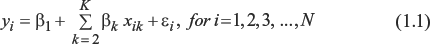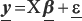This volume covers the commonly ignored topic of heteroskedasticity (unequal error variances) in regression analyses and provides a practical guide for how to proceed in terms of testing and correction. Emphasizing how to apply diagnostic tests and corrections for heteroskedasticity in actual data analyses, the book offers three approaches for dealing with heteroskedasticity:

variance-stabilizing transformations of the dependent variable; calculating robust standard errors, or heteroskedasticity-consistent standard errors; and; generalized least squares estimation coefficients and standard errors.

The detection and correction of heteroskedasticity is illustrated with three examples that vary in terms of sample size and the types of units analyzed (individuals, households, U.S. states). Intended as a supplementary text for graduate-level courses and a primer for quantitative researchers, the book fills the gap between the limited coverage of heteroskedasticity provided in applied regression textbooks and the more theoretical statistical treatment in advanced econometrics textbooks.

### What is Heteroskedasticity and Why should We Care?

For concreteness, consider the following linear regression model for a quantitative outcome (yi) determined by an intercept (β1), a set of predictors (x2, x3,…, xK) and their coefficients (β2, β3, … βJ), and a random error (εi):or in matrix notation,where y is an N × 1 column vector of the values of the outcome, X is an N × K matrix whose columns are the values of the predictors,1β is a 1 × K column vector of the coefficients, and ε is an N × 1 column vector of the values of the error term.

One of the usual ordinary least squares (OLS) assumptions is that the variance of εi is constant (see QASS # 50 by Berry ...

•••